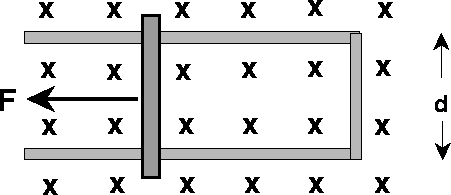# Faraday's Law: Conducting rod moving across a magnetic field

choco_moo

## Homework Statement

A conducting rod is pulled horizontally with constant force F= 3.40 N along a set of rails separated by d= 0.260 m. A uniform magnetic field B= 0.500T is directed into the page. There is no friction between the rod and the rails, and the rod moves with constant velocity v= 6.80 m/s.1. Using Faraday's Law, calculate the induced emf around the loop in the figure that is caused by the changing flux. Assign clockwise to be the positive direction for emf.

2. The emf around the loop causes a current to flow. How large is that current? (Again, use a positive value for clockwise direction.)

EMF = -d Φ/dt
Φ = ∫(B·dA)

## The Attempt at a Solution

1. I didn't expect this to work, but I tried to use the formula E = BLv. I plugged in B, d, and v and the answer was wrong.
A hint was given: When the magnetic field is uniform and normal to the plane of the loop, then the flux is of the product of the field and the area. In this problem it is the area that changes with time, not the field.
Even with the hint I'm still confused. Since area is changing with time, how do I incorporate that into my calculation?

2. I first tried using F = IL x B to solve for I but it was wrong. The hint said Remember F=ma: since v is constant, a=0, so it must be true that the net force on the rod is zero. The pulling force is compensating the force on the rod due to the current through it and the magnetic field.
So what does this mean? I'm so confused on what to do.

Any help is appreciated!

elect_eng
Any help is appreciated!

Honestly, it sounds to me that you are doing it correctly.

For part 1, the time derivative of the area will be $$d\; v$$. So why wouldn't the correct formula be $$EMF=B\; d\; v$$? The only place to make a mistake is with the sign, but the magnitude should be correct.

For part 2, normally the current would be the EMF divided by the resistance of the loop, but you do not know the resistance. However, you have correctly noted that the forces are balanced so the formula $$F=I \; d \; B$$ should enable you to calculate current $$I$$. You can even go one step further and calculate the resistance of the loop as $$R={{d^2\; B^2 \; v}\over {F}}$$

I don't know. Maybe we are both overlooking the same thing. Are you sure that your answer is wrong?

Something else bothers me here. Notice that the formula for resistance above shows the resistance is constant since d, B, v and F are all constant. However, if the perimeter of the loop is increasing, the resistance of the loop should also be increasing. Hence, it seems that you can not have a constant force balance as suggested in the problem. Again, maybe I'm overlooking something, and perhaps this last comment becomes a clue to the real solution. But, I must honestly say that I think there is an issue with the problem and with the answers you have been given.

EDIT: Although not explicitely stated in the problem, it seems that the rails are conductive, otherwise no current will flow.

Last edited:
choco_moo
Honestly, it sounds to me that you are doing it correctly.

For part 1, the time derivative of the area will be $$d\; v$$. So why wouldn't the correct formula be $$EMF=B\; d\; v$$? The only place to make a mistake is with the sign, but the magnitude should be correct.

For part 2, normally the current would be the EMF divided by the resistance of the loop, but you do not know the resistance. However, you have correctly noted that the forces are balanced so the formula $$F=I \; d \; B$$ should enable you to calculate current $$I$$. You can even go one step further and calculate the resistance of the loop as $$R={{d^2\; B^2 \; v}\over {F}}$$

I don't know. Maybe we are both overlooking the same thing. Are you sure that your answer is wrong?

Something else bothers me here. Notice that the formula for resistance above shows the resistance is constant since d, B, v and F are all constant. However, if the perimeter of the loop is increasing, the resistance of the loop should also be increasing. Hence, it seems that you can not have a constant force balance as suggested in the problem. Again, maybe I'm overlooking something, and perhaps this last comment becomes a clue to the real solution. But, I must honestly say that I think there is an issue with the problem and with the answers you have been given.

EDIT: Although not explicitely stated in the problem, it seems that the rails are conductive, otherwise no current will flow.

This time I tried using negatives for my answers and guess what, they're right! So this whole time, it was a sign problem. However, I don't really understand why they're negative. Can you explain why?